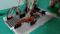Overview:

Let's play Monopoly! But we're using a different dice to roll. In this tutorial, we will build an Arduino-based two dice LED display. This is a simple project that utilizes the 8x8 Dot Matrix and the Tilt Sensor components found in the Arduino Kit Upgraded Starter Kit. A fun project that could be used in any situation that requires two dice.

This is project simulates two six-sided die that displays two random numbers when the tilt sensor is activated.

This tutorial explains how to use the 8x8 Dot Matrix, and the Tilt Sensor that comes with the kit. Previous knowledge of making functions will be utilized.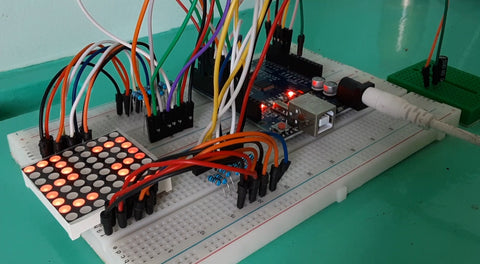Hardware Used:

• 8x8 LED Dot Matrix
•

• Tilt Sensor
• 12V 3A Power Supply
•

• 330Ω Resistor x 16
•

•

• Jumper Wires
•

Software Used:

• Arduino IDE

Application Discussion:

A dice is an instrument used to generate random numbers. The most common shape of a dice is a six-sided cube. It generates any number randomly from 1 to 6 by the action of throwing this instrument and acquiring the said random number on the top, face-up side of the dice. It is mostly used in tabletop games.

In this is project, we are going to be making an Arduino dice that simulates two six-sided die that displays two random numbers when the tilt sensor is activated for a game that uses two dice like Monopoly.

8x8 Dot Matrix LED Display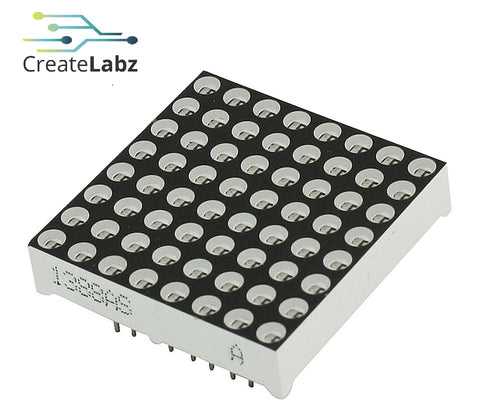The 8x8 dot matrix is an LED display arranged in 8 rows and 8 columns. It is controlled by 16 pins and each pin corresponds a row and a column number. The 8x8 dot matrix included in the kit is of the common anode configuration.

This component is wider than the common breadboard size so it is advised to use two breadboards joined together in order for the pins to be connected.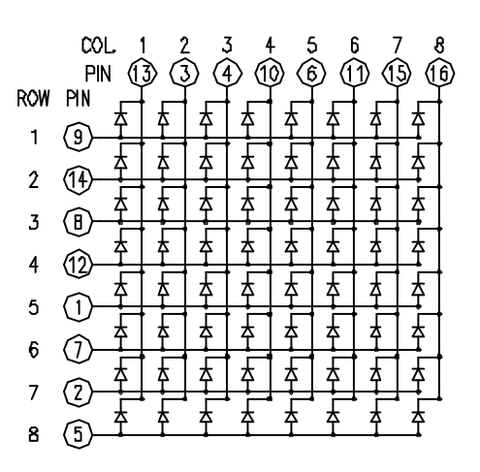Pin # Name Arduino 1 Row 5 6 2 Row 7 8 3 Col 2 11 4 Col 3 12 5 Row 8 9 6 Col 5 A0 7 Row 6 7 8 Row 3 4 9 Row 1 2 10 Col 4 13 11 Col 6 A1 12 Row 4 5 13 Col 1 10 14 Row 2 3 15 Col 7 A2 16 Col 8 A3
• Important Notes:

*Pin 1 orientation is on the side with the label and rounded knob as shown on the picture with the CreateLabz logo above

**Make sure to connect 330 Ohm or 220 Ohm resistors to the Dot Matrix pins in series with the Arduino pins

Tilt Sensor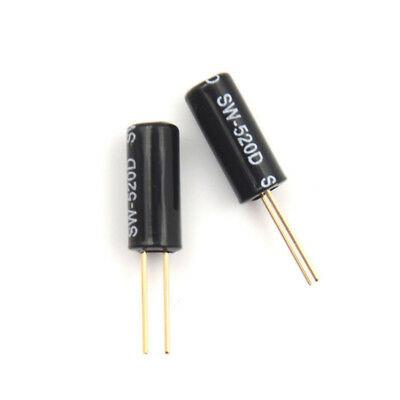The tilt sensor detects vibration and directional changes. This sensor has tiny metal balls inside that rolls around when the orientation of the component is changed.

The metal balls connect the pins, making the sensor emit a HIGH state when read by the Arduino. When the sensor is flipped upside down, the connection between pins is lost, thus emitting a LOW reading when read by the Arduino.

One pin is connected to one of the analog pins and the other is connected directly to GND.

Hardware Setup:

This is the breadboard configuration of the system. All parts are included in the Arduino Upgraded Starter Kit.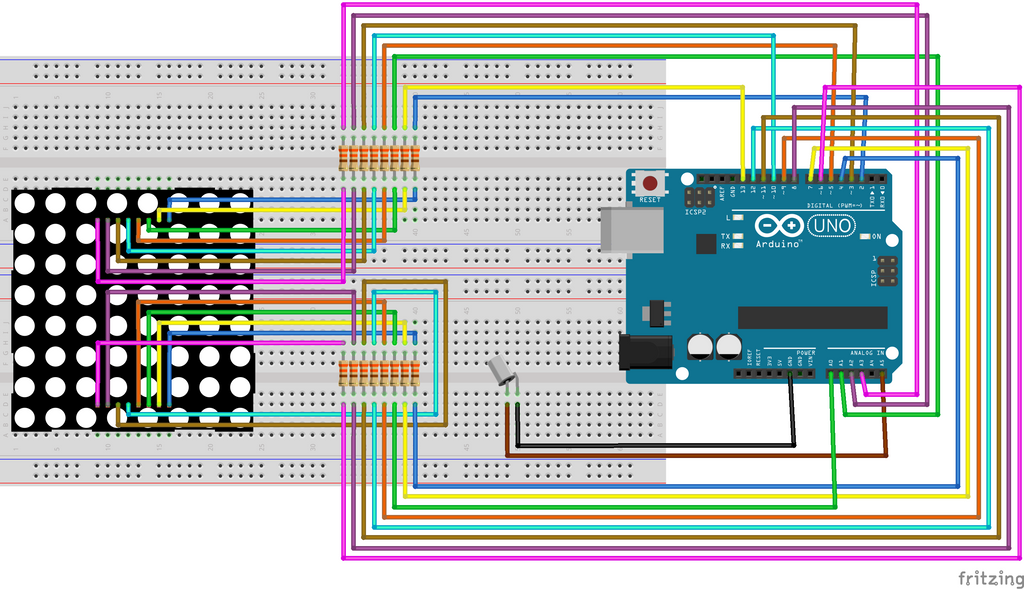The tilt sensor could be applied on a mini breadboard or female-male jumper wires to make tilting easier.

Software Setup:

Open the Arduino IDE and upload the code on the Arduino Uno Board.

Code:

The following is the Arduino code. You can download the code in our GitHub page here or copy the code below.
``````#define sensorPin A5

#define ROW_1 2
#define ROW_2 3
#define ROW_3 4
#define ROW_4 5
#define ROW_5 6
#define ROW_6 7
#define ROW_7 8
#define ROW_8 9

#define COL_1 10
#define COL_2 11
#define COL_3 12
#define COL_4 13
#define COL_5 A0
#define COL_6 A1
#define COL_7 A2
#define COL_8 A3

const byte rows[] = {
ROW_1, ROW_2, ROW_3, ROW_4, ROW_5, ROW_6, ROW_7, ROW_8
};
const byte col[] = {
COL_1,COL_2, COL_3, COL_4, COL_5, COL_6, COL_7, COL_8
};

int sensorValue;
int lastTiltState = HIGH;
long lastDebounceTime = 0;
long debounceDelay = 50;

int num1;
int num2;

//dice combinations
byte oneone[] = {
B01000000,
B11000010,
B01000110,
B01000010,
B01000010,
B01000010,
B11100010,
B00000111
};
byte onetwo[] = {
B01000000,
B11000111,
B01000001,
B01000001,
B01000111,
B01000100,
B11100100,
B00000111
};
byte onethree[] = {
B01000000,
B11000111,
B01000001,
B01000001,
B01000111,
B01000001,
B11100001,
B00000111
};
byte onefour[] = {
B01000000,
B11000001,
B01000011,
B01000101,
B01000111,
B01000001,
B11100001,
B00000001
};
byte onefive[] = {
B01000000,
B11000111,
B01000100,
B01000100,
B01000111,
B01000001,
B11100001,
B00000111
};
byte onesix[] = {
B01000000,
B11000111,
B01000100,
B01000100,
B01000111,
B01000101,
B11100101,
B00000111
};
byte twoone[] = {
B11100000,
B00100010,
B00100110,
B11100010,
B10000010,
B10000010,
B11100010,
B00000111
};
byte twotwo[] = {
B11100000,
B00100111,
B00100001,
B11100001,
B10000111,
B10000100,
B11100100,
B00000111
};
byte twothree[] = {
B11100000,
B00100111,
B00100001,
B11100001,
B10000111,
B10000001,
B11100001,
B00000111
};
byte twofour[] = {
B11100000,
B00100001,
B00100011,
B11100101,
B10000111,
B10000001,
B11100001,
B00000001
};
byte twofive[] = {
B11100000,
B00100111,
B00100100,
B11100100,
B10000111,
B10000001,
B11100001,
B00000111
};
byte twosix[] = {
B11100000,
B00100111,
B00100100,
B11100100,
B10000111,
B10000101,
B11100101,
B00000111
};
byte threeone[] = {
B11100000,
B00100010,
B00100110,
B11100010,
B00100010,
B00100010,
B11100010,
B00000111
};
byte threetwo[] = {
B11100000,
B00100111,
B00100001,
B11100001,
B00100111,
B00100100,
B11100100,
B00000111
};
byte threethree[] = {
B11100000,
B00100111,
B00100001,
B11100001,
B00100111,
B00100001,
B11100001,
B00000111
};
byte threefour[] = {
B11100000,
B00100001,
B00100011,
B11100101,
B00100111,
B00100001,
B11100001,
B00000001
};
byte threefive[] = {
B11100000,
B00100111,
B00100100,
B11100100,
B00100111,
B00100001,
B11100001,
B00000111
};
byte threesix[] = {
B11100000,
B00100111,
B00100100,
B11100100,
B00100111,
B00100101,
B11100101,
B00000111
};
byte fourone[] = {
B00100000,
B01100010,
B10100110,
B11100010,
B00100010,
B00100010,
B00100010,
B00000111
};
byte fourtwo[] = {
B00100000,
B01100111,
B10100001,
B11100001,
B00100111,
B00100100,
B00100100,
B00000111
};
byte fourthree[] = {
B00100000,
B01100111,
B10100001,
B11100001,
B00100111,
B00100001,
B00100001,
B00000111
};
byte fourfour[] = {
B00100000,
B01100111,
B10100100,
B11100100,
B00100111,
B00100001,
B00100001,
B00000111
};
byte fourfive[] = {
B00100000,
B01100111,
B10100100,
B11100100,
B00100111,
B00100101,
B00100101,
B00000111
};
byte foursix[] = {
B11100000,
B10000010,
B10000110,
B11100010,
B00100010,
B00100010,
B11100010,
B00000111
};
byte fiveone[] = {
B11100000,
B10000111,
B10000001,
B11100001,
B00100111,
B00100100,
B11100100,
B00000111
};
byte fivetwo[] = {
B11100000,
B10000111,
B10000001,
B11100001,
B00100111,
B00100001,
B11100001,
B00000111
};
byte fivethree[] = {
B11100000,
B10000001,
B10000011,
B11100101,
B00100111,
B00100001,
B11100001,
B00000001
};
byte fivefour[] = {
B11100000,
B10000111,
B10000100,
B11100100,
B00100111,
B00100001,
B11100001,
B00000111
};
byte fivefive[] = {
B11100000,
B10000111,
B10000100,
B11100100,
B00100111,
B00100101,
B11100101,
B00000111
};
byte fivesix[] = {
B01000000,
B11000010,
B01000110,
B01000010,
B01000010,
B01000010,
B11100010,
B00000111
};
byte sixone[] = {
B11100000,
B10000010,
B10000110,
B11100010,
B10100010,
B10100010,
B11100010,
B00000111
};
byte sixtwo[] = {
B11100000,
B10000111,
B10000001,
B11100001,
B10100111,
B10100100,
B11100100,
B00000111
};
byte sixthree[] = {
B11100000,
B10000111,
B10000001,
B11100001,
B10100111,
B10100001,
B11100001,
B00000111
};
byte sixfour[] = {
B11100000,
B10000001,
B10000011,
B11100101,
B10100111,
B10100001,
B11100001,
B00000001
};
byte sixfive[] = {
B11100000,
B10000111,
B10000100,
B11100100,
B10100111,
B10100001,
B11100001,
B00000111
};
byte sixsix[] = {
B11100000,
B10000111,
B10000100,
B11100100,
B10100111,
B10100101,
B11100101,
B00000111
};

void setup() {
pinMode(sensorPin, INPUT);
digitalWrite(sensorPin, HIGH);

for (byte i = 2; i <= 13; i++){
pinMode(i, OUTPUT);
}
pinMode(A0, OUTPUT);
pinMode(A1, OUTPUT);
pinMode(A2, OUTPUT);
pinMode(A3, OUTPUT);
}

void loop() {

if (sensorValue == lastTiltState) {
//reset the debouncing timer
lastDebounceTime = millis();
num1 = random(1,6);
num2 = random(1,6);
}

//when the sensor orientation changes
if (sensorValue != lastTiltState) {
diceDisplay();
}

if ((millis() - lastDebounceTime) > debounceDelay) {
lastTiltState = sensorValue;
}

delay(500);
}

void diceDisplay() {
if (num1 == 1 && num2 == 1) {
for(int i = 0 ; i < 100 ; i++)        //Loop display 100 times
{
Display(oneone);
}
}
if (num1 == 1 && num2 == 2) {
for(int i = 0 ; i < 100 ; i++)
{
Display(onetwo);
}
}
if (num1 == 1 && num2 == 3) {
for(int i = 0 ; i < 100 ; i++)
{
Display(onethree);
}
}
if (num1 == 1 && num2 == 4) {
for(int i = 0 ; i < 100 ; i++)
{
Display(onefour);
}
}
if (num1 == 1 && num2 == 5) {
for(int i = 0 ; i < 100 ; i++)
{
Display(onefive);
}
}
if (num1 == 1 && num2 == 6) {
for(int i = 0 ; i < 100 ; i++)
{
Display(onesix);
}
}
if (num1 == 2 && num2 == 1) {
for(int i = 0 ; i < 100 ; i++)
{
Display(twoone);
}
}
if (num1 == 2 && num2 == 2) {
for(int i = 0 ; i < 100 ; i++)
{
Display(twotwo);
}
}
if (num1 == 2 && num2 == 3) {
for(int i = 0 ; i < 100 ; i++)
{
Display(twothree);
}
}
if (num1 == 2 && num2 == 4) {
for(int i = 0 ; i < 100 ; i++)
{
Display(twofour);
}
}
if (num1 == 2 && num2 == 5) {
for(int i = 0 ; i < 100 ; i++)
{
Display(twofive);
}
}
if (num1 == 2 && num2 == 6) {
for(int i = 0 ; i < 100 ; i++)
{
Display(twosix);
}
}
if (num1 == 3 && num2 == 1) {
for(int i = 0 ; i < 100 ; i++)
{
Display(threeone);
}
}
if (num1 == 3 && num2 == 2) {
for(int i = 0 ; i < 100 ; i++)
{
Display(threetwo);
}
}
if (num1 == 3 && num2 == 3) {
for(int i = 0 ; i < 100 ; i++)
{
Display(threethree);
}
}
if (num1 == 3 && num2 == 4) {
for(int i = 0 ; i < 100 ; i++)
{
Display(threefour);
}
}
if (num1 == 3 && num2 == 5) {
for(int i = 0 ; i < 100 ; i++)
{
Display(threefive);
}
}
if (num1 == 3 && num2 == 6) {
for(int i = 0 ; i < 100 ; i++)
{
Display(threesix);
}
}
if (num1 == 4 && num2 == 1) {
for(int i = 0 ; i < 100 ; i++)
{
Display(fourone);
}
}
if (num1 == 4 && num2 == 2) {
for(int i = 0 ; i < 100 ; i++)
{
Display(fourtwo);
}
}
if (num1 == 4 && num2 == 3) {
for(int i = 0 ; i < 100 ; i++)
{
Display(fourthree);
}
}
if (num1 == 4 && num2 == 4) {
for(int i = 0 ; i < 100 ; i++)
{
Display(fourfour);
}
}
if (num1 == 4 && num2 == 5) {
for(int i = 0 ; i < 100 ; i++)
{
Display(fourfive);
}
}
if (num1 == 4 && num2 == 6) {
for(int i = 0 ; i < 100 ; i++)
{
Display(foursix);
}
}
if (num1 == 5 && num2 == 1) {
for(int i = 0 ; i < 100 ; i++)
{
Display(fiveone);
}
}
if (num1 == 5 && num2 == 2) {
for(int i = 0 ; i < 100 ; i++)
{
Display(fivetwo);
}
}
if (num1 == 5 && num2 == 3) {
for(int i = 0 ; i < 100 ; i++)
{
Display(fivethree);
}
}
if (num1 == 5 && num2 == 4) {
for(int i = 0 ; i < 100 ; i++)
{
Display(fivefour);
}
}
if (num1 == 5 && num2 == 5) {
for(int i = 0 ; i < 100 ; i++)
{
Display(fivefive);
}
}
if (num1 == 5 && num2 == 6) {
for(int i = 0 ; i < 100 ; i++)
{
Display(fivesix);
}
}
if (num1 == 6 && num2 == 1) {
for(int i = 0 ; i < 100 ; i++)
{
Display(sixone);
}
}
if (num1 == 6 && num2 == 2) {
for(int i = 0 ; i < 100 ; i++)
{
Display(sixtwo);
}
}
if (num1 == 6 && num2 == 3) {
for(int i = 0 ; i < 100 ; i++)
{
Display(sixthree);
}
}
if (num1 == 6 && num2 == 4) {
for(int i = 0 ; i < 100 ; i++)
{
Display(sixfour);
}
}
if (num1 == 6 && num2 == 5) {
for(int i = 0 ; i < 100 ; i++)
{
Display(sixfive);
}
}
if (num1 == 6 && num2 == 6) {
for(int i = 0 ; i < 100 ; i++)
{
Display(sixsix);
}
}
}

void  Display(byte buffer2[]){
// Turn on each row in series
for (byte i = 0; i < 8; i++) {
setCols(buffer2[i]);

digitalWrite(rows[i], HIGH);
delay(1);
digitalWrite(rows[i], LOW);
delay(1);
}
}

void setCols(byte b) {
digitalWrite(COL_1, (~b >> 0) & 0x01); // Get the 1st bit: 10000000
digitalWrite(COL_2, (~b >> 1) & 0x01); // Get the 2nd bit: 01000000
digitalWrite(COL_3, (~b >> 2) & 0x01); // Get the 3rd bit: 00100000
digitalWrite(COL_4, (~b >> 3) & 0x01); // Get the 4th bit: 00010000
digitalWrite(COL_5, (~b >> 4) & 0x01); // Get the 5th bit: 00001000
digitalWrite(COL_6, (~b >> 5) & 0x01); // Get the 6th bit: 00000100
digitalWrite(COL_7, (~b >> 6) & 0x01); // Get the 7th bit: 00000010
digitalWrite(COL_8, (~b >> 7) & 0x01); // Get the 8th bit: 00000001
}
``````

Code Breakdown:

• #define construct

The #define component used at the start of the code is used to define constants that could not be changed when the program is running. It is very useful in this application because it does not use any program memory on the chip.

Make sure to use this when assigning Arduino GPIO pins so it won't use up your memory.

• Dice Combinations
The dice combinations set on byte arrays are made using this LED Matrix Editor. This is especially important since byte arrays eats up much less memory than char arrays.
 ``````byte oneone[] = { B01000000, B11000010, B01000110, B01000010, B01000010, B01000010, B11100010, B00000111 }; ``````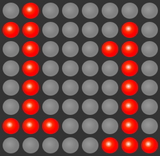Look at the byte array closely and you can see that the LED is lit up at the 1's and off at the 0's. You can design your own without the editor by inputting the LEDs you want lit with 1's.

The byte array that is automatically generated by the editor could be found on the left side of the screen.

• setup()

In this function, the pins used are set as INPUT for the sensor and OUTPUT for the LED matrix pins.

The digitalWrite(sensorPin, HIGH) with sensorPIn set as INPUT is very important since this project does not use a 10k ohm pull-up resistor for the tilt sensor. Setting the sensorPin to HIGH uses the Arduino's internal resistance instead of using an extra component. Especially handy when saving resources.

• loop()

Any commands set here will be executed indefinitely. This function reads the sensor input for any changes. When it is not tilted, the following loop is run:

``````if (sensorValue == lastTiltState) {
// reset the debouncing timer
lastDebounceTime = millis();
num1 = random(1,6);
num2 = random(1,6);
}``````

The random(min,max) function is called. This is a function built in the Arduino that chooses a random number within the boundaries set by the user. In this case, a number is to be randomly chosen from 1 to 6 like a dice, and stores it at variables num1 and num2.

However, when the sensor is tilted, the following loop is run:

``if (sensorValue != lastTiltState) { diceDisplay();}``

This condition runs the diceDiplay() function.

• Display()
This function turns on every row one by one for about 1 millisecond (very fast!) each. It calls on setCols() function as it loops through each row.

• setCols()

This function is called to check the byte array and identify which columns need to be lit (the ones identified with 1's in the byte array).

Video Demo:

Conclusion:

The Arduino dice is a fun project that could be used in games that are to be played with friends and family. Not only is this fun, it is also educational. This project was able to show how to drive an 8x8 Dot Matrix using an Arduino, how to save memory by using the #define component, and how to utilize the 'built-in' pull-up resistor by using digitalWrite().

To find more useful projects, continue reading the Knowledgebase and Tutorials.

References:

https://www.instructables.com/8x8-LED-Matrix-Using-Arduino